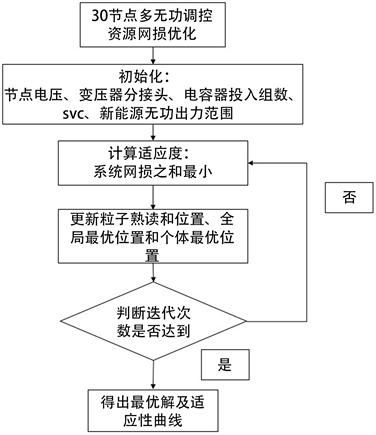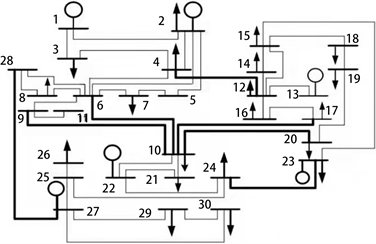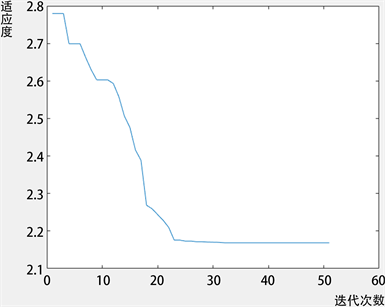#### 期刊菜单

Optimization Method for Multi-Reactive Power Regulation Resources of Wind Power-Containing Systems Based on Particle Swarm Algorithm
DOI: 10.12677/SG.2022.123008, PDF, HTML, XML, 下载: 174  浏览: 267  科研立项经费支持

Abstract: As a high-quality renewable energy power generation technology, wind power generation can not only alleviate energy shortage, but also improve environmental pollution. However, with the increase of the proportion of wind power connected to the grid, the network loss of wind power system increases sharply. In this paper, taking the minimum active power network loss of the system as the objective function and the conventional generator set, wind turbine set, static reactive power compensator, capacitor reactor and transformer tap as the reactive power control resources, the mathematical model of reactive power optimization control of wind power system is established, and the particle swarm optimization algorithm is used to solve the model. Finally, based on MATLAB platform, the established mathematical model is analyzed in the IEEE30 node system after wind power grid connection. The results show that this method is effective and feasible in reducing the network loss of wind power system.

1. 引言

2. 多无功调控资源的优化控制模型

2.1. 多无功调控资源能力分析Table 1. Comparison of multi-reactive power control resource performance

2.2. 多无功源优化控制模型

2.2.1. 目标函数

$\mathrm{min}\Delta {P}_{\sum }=\underset{i=1}{\overset{i=N}{\sum }}{P}_{Gi}+\underset{i=1}{\overset{i=M}{\sum }}{P}_{Wi}-\underset{i=1}{\overset{i=K}{\sum }}{P}_{Li}$ (1)

2.2.2. 约束条件

${U}_{i}\underset{j=1}{\overset{j=n}{\sum }}{U}_{j}\left({G}_{ij}\mathrm{cos}{\delta }_{ij}+{B}_{ij}\mathrm{sin}{\delta }_{ij}\right)={P}_{i}$ (2)

${U}_{i}\underset{j=1}{\overset{j=n}{\sum }}{U}_{j}\left({G}_{ij}\mathrm{sin}{\delta }_{ij}+{B}_{ij}\mathrm{cos}{\delta }_{ij}\right)={Q}_{i}$ (3)

$\left\{\begin{array}{c}{P}_{Gi\mathrm{min}}\le {P}_{Gi}\le {P}_{Gi\mathrm{max}}\\ {Q}_{Gi\mathrm{min}}\le {Q}_{Gi}\le {Q}_{Gi\mathrm{max}}\\ {P}_{Wi\mathrm{min}}\le {P}_{Wi}\le {P}_{Wi\mathrm{max}}\\ {Q}_{Wi\mathrm{min}}\le {Q}_{Wi}\le {Q}_{Wi\mathrm{max}}\\ {U}_{i\mathrm{min}}\le {U}_{i}\le {U}_{i\mathrm{max}}\\ {T}_{h\mathrm{min}}\le {T}_{h}\le {T}_{h\mathrm{max}}\\ {Q}_{Ci\mathrm{min}}\le {Q}_{Ci}\le {Q}_{Ci\mathrm{max}}\\ {Q}_{SVCi\mathrm{min}}\le {Q}_{SVCi}\le {Q}_{SVCi\mathrm{max}}\end{array}$ (4)

$|{\delta }_{i}-{\delta }_{j}|<\mathrm{max}|{\delta }_{i}-{\delta }_{j}|$

3. 基于粒子群算法的多无功调控资源优化控制方法

3.1. 粒子群算法基本原理Figure 1. Optimization control flowchart of multi-reactive power control resource based on particle swarm algorithm

${v}_{id}^{t+1}=\omega {v}_{id}^{t}+{c}_{1}{r}_{1}\left({P}_{best}-{x}_{id}^{t}\right)+{c}_{2}{r}_{2}\left({G}_{best}-{x}_{id}^{t}\right)$

${x}_{id}^{t+1}={x}_{id}^{t}+{v}_{id}^{t+1}$

3.2. 多无功调控资源优化控制方法

4. 算例分析

4.1. 算例设置Figure 2. IEEE30 node diagram

4.2. 算例结果分析Table 2. Power plant node parametersTable 4. Power plant node optimization results (Mvar)Table 5. Optimization results of ordinary nodes (nominal values)Table 6. Active network loss optimization results (MW)Figure 3. Fitness curve chart

5. 总结

  陈珩, 编. 电力系统稳态分析[M]. 北京: 中国电力出版社, 2015.  简献忠, 李莹, 范建鹏, 柏勰文, 杨延安. 基于差分进化粒子群算法的多目标无功优化[J]. 控制工程, 2015(1): 113-117.  李佳, 刘天琪, 李兴源, 邢大鹏, 李茜, 江东林, 肖军. 改进粒子群-禁忌搜索算法在多目标无功优化中的应用[J]. 电力自动化设备, 2014, 34(8): 71-77.  王军昌. 风能发电和火力发电的发展潜力对比研究[J]. 电子制作, 2015(2): 232.  任振宇, 张师, 周毅博. 大规模风电并网系统的无功优化控制方法[J]. 智能电网, 2017(3): 250-253.  胡艺文. 基于新能源并网的电站综合无功优化控制算法研究[J]. 电测与仪表, 2015(22): 90-94.  陶志东, 顾浩, 丁晓群. 基于电压稳定影响的多目标无功优化研究[J]. 电网分析与研究, 2018, 46(10): 63-70.  张俊, 张安安. IEEE30节点系统电压/无功优化[J]. 物联网技术, 2016(5): 43-48.  石江浩, 李锴, 张师. 基于PSO的风电并网系统多时段无功优化控制[J]. 电气开关, 2020(4): 44-50.  柯国华, 陈新河, 周琪, 陈芳雨. 电力系统无功优化的算法概述[J]. 机电信息, 2022(1): 83-85. https://doi.org/10.19514/j.cnki.cn32-1628/tm.2022.01.023  袁晓辉, 王乘, 张勇传, 袁艳斌. 粒子群优化算法在电力系统中的应用[J]. 电网技术, 2004(19): 14-19.